x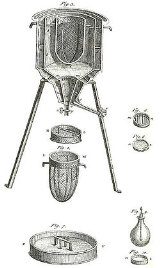CalorimeterOverview

A calorimeter is a device used for calorimetry
Calorimetry
Calorimetry is the science of measuring the heat of chemical reactions or physical changes. Calorimetry is performed with a calorimeter. The word calorimetry is derived from the Latin word calor, meaning heat...

, the science
Science
Science is a systematic enterprise that builds and organizes knowledge in the form of testable explanations and predictions about the universe...

of measuring the heat of chemical reaction
Chemical reaction
A chemical reaction is a process that leads to the transformation of one set of chemical substances to another. Chemical reactions can be either spontaneous, requiring no input of energy, or non-spontaneous, typically following the input of some type of energy, such as heat, light or electricity...

s or physical change
Physical change
Physical changes are changes affecting the form of a chemical substance, but do not change the chemical composition of that substance. Physical changes are used to separate mixtures into their component compounds, but can not usually be used to separate compounds into chemical elements or simpler...

s as well as heat capacity
Heat capacity
Heat capacity , or thermal capacity, is the measurable physical quantity that characterizes the amount of heat required to change a substance's temperature by a given amount...

. Differential scanning calorimeters, isothermal microcalorimeters, titration calorimeters and accelerated rate calorimeters are among the most common types. A simple calorimeter just consists of a thermometer attached to a metal container full of water suspended above a combustion chamber.

To find the enthalpy
Enthalpy
Enthalpy is a measure of the total energy of a thermodynamic system. It includes the internal energy, which is the energy required to create a system, and the amount of energy required to make room for it by displacing its environment and establishing its volume and pressure.Enthalpy is a...

change per mole
Mole (unit)
The mole is a unit of measurement used in chemistry to express amounts of a chemical substance, defined as an amount of a substance that contains as many elementary entities as there are atoms in 12 grams of pure carbon-12 , the isotope of carbon with atomic weight 12. This corresponds to a value...

of a substance A in a reaction between two substances A and B, the substances are added to a calorimeter and the initial and final temperature
Temperature
Temperature is a physical property of matter that quantitatively expresses the common notions of hot and cold. Objects of low temperature are cold, while various degrees of higher temperatures are referred to as warm or hot...

s (before the reaction started and after it has finished) are noted.Unanswered QuestionsHow does one measure calories in liquids (specifically, soda/softdrinks) using a calorimeter?How calorimeter is used to find energy levels of food?A calorimeter cup was standardized using 40.00 mL of 1.00 M HCl and 80.00 mL of 0.500 M M(OH)2. The neutralization reaction gave a temperature rise o...what type of calorimeter is used in this experimeter, constant pressure or constant volume?Encyclopedia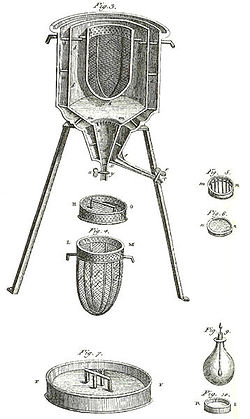A calorimeter is a device used for calorimetry
Calorimetry
Calorimetry is the science of measuring the heat of chemical reactions or physical changes. Calorimetry is performed with a calorimeter. The word calorimetry is derived from the Latin word calor, meaning heat...

, the science
Science
Science is a systematic enterprise that builds and organizes knowledge in the form of testable explanations and predictions about the universe...

of measuring the heat of chemical reaction
Chemical reaction
A chemical reaction is a process that leads to the transformation of one set of chemical substances to another. Chemical reactions can be either spontaneous, requiring no input of energy, or non-spontaneous, typically following the input of some type of energy, such as heat, light or electricity...

s or physical change
Physical change
Physical changes are changes affecting the form of a chemical substance, but do not change the chemical composition of that substance. Physical changes are used to separate mixtures into their component compounds, but can not usually be used to separate compounds into chemical elements or simpler...

s as well as heat capacity
Heat capacity
Heat capacity , or thermal capacity, is the measurable physical quantity that characterizes the amount of heat required to change a substance's temperature by a given amount...

. Differential scanning calorimeters, isothermal microcalorimeters, titration calorimeters and accelerated rate calorimeters are among the most common types. A simple calorimeter just consists of a thermometer attached to a metal container full of water suspended above a combustion chamber.

To find the enthalpy
Enthalpy
Enthalpy is a measure of the total energy of a thermodynamic system. It includes the internal energy, which is the energy required to create a system, and the amount of energy required to make room for it by displacing its environment and establishing its volume and pressure.Enthalpy is a...

change per mole
Mole (unit)
The mole is a unit of measurement used in chemistry to express amounts of a chemical substance, defined as an amount of a substance that contains as many elementary entities as there are atoms in 12 grams of pure carbon-12 , the isotope of carbon with atomic weight 12. This corresponds to a value...

of a substance A in a reaction between two substances A and B, the substances are added to a calorimeter and the initial and final temperature
Temperature
Temperature is a physical property of matter that quantitatively expresses the common notions of hot and cold. Objects of low temperature are cold, while various degrees of higher temperatures are referred to as warm or hot...

s (before the reaction started and after it has finished) are noted. Multiplying the temperature change by the mass and specific heat capacities of the substances gives a value for the energy
Energy
In physics, energy is an indirectly observed quantity. It is often understood as the ability a physical system has to do work on other physical systems...

given off or absorbed during the reaction. Dividing the energy change by how many moles of A were present gives its enthalpy change of reaction. This method is used primarily in academic teaching as it describes the theory of calorimetry. It does not account for the heat loss through the container or the heat capacity of the thermometer and container itself. In addition, the object placed inside the calorimeter show that the objects transferred their heat to the calorimeter and into the liquid, and the heat absorbed by the calorimeter and the liquid is equal to the heat given off by the metals.

## Adiabatic calorimeters

An adiabatic
Adiabatic process
In thermodynamics, an adiabatic process or an isocaloric process is a thermodynamic process in which the net heat transfer to or from the working fluid is zero. Such a process can occur if the container of the system has thermally-insulated walls or the process happens in an extremely short time,...

calorimeter is a calorimeter used to examine a runaway reaction. Since the calorimeter runs in an adiabatic environment, any heat generated by the material sample under test causes the sample to increase in temperature, thus fuelling the reaction.

No adiabatic calorimeter is truly adiabatic - some heat will be lost by the sample to the sample holder. A mathematical correction factor, known as the phi-factor, can be used to adjust the calorimetric result to account for these heat losses. The phi-factor is the ratio of the thermal mass of the sample holder to the thermal mass of the sample alone.
Examples of adiabatic calorimeters are:-
• THT EV-Accelerating Rate Calorimeter
• HEL Phi-Tec
• A simple Dewar flask
Dewar flask
A Dewar flask is a vessel designed to provide very good thermal insulation. For instance, when filled with a hot liquid, the vessel will not allow the heat to easily escape, and the liquid will stay hot for far longer than in a typical container...

• Systag FlexyTSC a successor of their SIKAREX unit - the electronics of which could be used to apply a feedback system to heat the sample holder to give a result closer to true adiabaticy, however as the sample holder is an open ended glass tube, one soon loses the sample as a great deal of smoke.

## Reaction calorimeters

A reaction calorimeter is a calorimeter in which a chemical reaction
Chemical reaction
A chemical reaction is a process that leads to the transformation of one set of chemical substances to another. Chemical reactions can be either spontaneous, requiring no input of energy, or non-spontaneous, typically following the input of some type of energy, such as heat, light or electricity...

is initiated within a closed insulated container. Reaction heats are measured and the total heat is obtained by integrating heatflow versus time. This is the standard used in industry to measure heats since industrial processes are engineered to run at constant temperatures. Reaction calorimetry can also be used to determine maximum heat release rate for chemical process engineering and for tracking the global kinetics of reactions. There are four main methods for measuring the heat in reaction calorimeter:

### Heat flow calorimetry

The cooling/heating jacket controls either the temperature of the process or the temperature of the jacket. Heat is measured by monitoring the temperature difference between heat transfer fluid and the process fluid. In addition fill volumes (i.e. wetted area), specific heat, heat transfer coefficient have to be determined to arrive at a correct value. It is possible with this type of calorimeter to do reactions at reflux, although the accuracy is not as good.

### Heat balance calorimeter

The cooling/heating jacket controls the temperature of the process. Heat is measured by monitoring the heat gained or lost by the heat transfer fluid.

### Power compensation

Power compensation uses a heater placed within the vessel to maintain a constant temperature. The energy supplied to this heater can be varied as reactions require and the calorimetry signal is purely derived from this electrical power.

### Constant flux

Constant flux calorimetry (or COFLUX as it is often termed) is derived from heat balance calorimetry and uses specialized control mechanisms to maintain a constant heat flow (or flux) across the vessel wall.

## Bomb calorimeters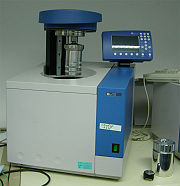A bomb calorimeter is a type of constant-volume calorimeter used in measuring the heat of combustion of a particular reaction. Bomb calorimeters have to withstand the large pressure within the calorimeter as the reaction is being measured. Electrical energy is used to ignite the fuel; as the fuel is burning, it will heat up the surrounding air, which expands and escapes through a tube that leads the air out of the calorimeter. When the air is escaping through the copper tube it will also heat up the water outside the tube. The temperature of the water allows for calculating calorie content of the fuel.

In more recent calorimeter designs, the whole bomb, pressurized with excess pure oxygen (typically at 30atm) and containing a known mass of sample (typically 1-1.5 g) and a small fixed amount of water (to absorb produced acid gases), is submerged under a known volume of water (ca. 2000 ml) before the charge is (again electrically) ignited. The bomb, with sample and oxygen, form a closed system - no air escapes during the reaction. The energy released by the combustion raises the temperature of the steel bomb, its contents, and the surrounding water jacket. The temperature change in the water is then accurately measured. This temperature rise, along with a bomb factor (which is dependent on the heat capacity of the metal bomb parts) is used to calculate the energy given out by the sample burn. A small correction is made to account for the electrical energy input, the burning fuse, and acid production (by titration of the residual liquid). After the temperature rise has been measured, the excess pressure in the bomb is released.

Basically, a bomb calorimeter consists of a small cup to contain the sample, oxygen, a stainless steel bomb, water, a stirrer, a thermometer, the dewar (to prevent heat flow from the calorimeter to the surroundings) and ignition circuit connected to the bomb.

Since there is no heat exchange between the calorimeter and surroundings → Q = 0 (adiabatic) ; no work performed → W = 0
Thus, the total internal energy change ΔU(total) = Q + W = 0

Also, total internal energy change ΔU(total) = ΔU(system) + ΔU(surroundings) = 0
→ ΔU(system) = - ΔU(surroundings) = -Cv ΔT (constant volume → dV = 0)

where Cv = heat capacity of the bomb

Before the bomb can be used to determine heat of combustion of any compound, it must be calibrated.
The value of Cv can be estimated by
Cv (calorimeter) = m (water). Cv (water) + m (steel). Cv (steel)

m (water) and m (steel) can be measured;

Cv(water)= 1 cal/g.K

Cv(steel)= 0.1 cal/g.K

In laboratory, Cv is determined by running a compound with known heat of combustion value: Cv = Hc/ΔT

Common compounds are benzoic acid
Benzoic acid
Benzoic acid , C7H6O2 , is a colorless crystalline solid and the simplest aromatic carboxylic acid. The name derived from gum benzoin, which was for a long time the only source for benzoic acid. Its salts are used as a food preservative and benzoic acid is an important precursor for the synthesis...

(Hc = 6318 cal/g) or p-methyl benzoic acid (Hc = 6957 cal/g).

Temperature (T) is recorded every minute and ΔT = T(final) - T(initial)

A small factor contributes to the correction of the total heat of combustion is the fuse wire. Nickel fuse wire is often used and has heat of combustion = 981.3 cal/g

In order to calibrate the bomb, a small amount (~ 1 g) of benzoic acid, or p-methyl benzoic acid is weighed.
A length of Nickel fuse wire (~10 cm) is weighed both before and after the combustion process. Mass of fuse wire burned Δm = m(before) - m(after)

The combustion of sample (benzoic acid) inside the bomb ΔHc = ΔHc (benzoic acid) x m (benzoic acid) + ΔHc (Ni fuse wire) x Δm (Ni fuse wire)

ΔHc = Cv. ΔT → Cv = ΔHc/ΔT

Once Cv value of the bomb is determined, the bomb is ready to use to calculate heat of combustion of any compounds by ΔHc = Cv. ΔT

## Calvet-type calorimeters

The detection is based on a three-dimensional fluxmeter sensor. The fluxmeter element consists of a ring of several thermocouples in series. The corresponding thermopile of high thermal conductivity surrounds the experimental space within the calorimetric block. The radial arrangement of the thermopiles guarantees an almost complete integration of the heat. This is verified by the calculation of the efficiency ratio that indicates that an average value of 94 % +/- 1 % of heat is transmitted through the sensor on the full range of temperature of the Calvet-type calorimeter. In this setup, the sensitivity of the calorimeter is not affected by the crucible, the type of purgegas, or the flow rate. The main advantage of the setup is the increase of the experimental vessel's size and consequently the size of the sample, without affecting the accuracy of the calorimetric measurement.

The calibration of the calorimetric detectors is a key parameter and has to be performed very carefully. For Calvet-type calorimeters, a specific calibration, so called Joule effect or electrical calibration, has been developed to overcome all the problems encountered by a calibration done with standard materials.
The main advantages of this type of calibration are as follows:
• It is an absolute calibration.
• The use of standard materials for calibration is not necessary. The calibration can be performed at a constant temperature, in the heating mode and in the cooling mode.
• It can be applied to any experimental vessel volume.
• It is a very accurate calibration.

An example of Calvet-type calorimeter is the C80 Calorimeter (reaction, isothermal and scanning calorimeter).

## Constant-pressure calorimeter

A constant-pressure calorimeter measures the change in enthalpy
Enthalpy
Enthalpy is a measure of the total energy of a thermodynamic system. It includes the internal energy, which is the energy required to create a system, and the amount of energy required to make room for it by displacing its environment and establishing its volume and pressure.Enthalpy is a...

of a reaction occurring in solution
Solution
In chemistry, a solution is a homogeneous mixture composed of only one phase. In such a mixture, a solute is dissolved in another substance, known as a solvent. The solvent does the dissolving.- Types of solutions :...

during which the atmospheric pressure
Atmospheric pressure
Atmospheric pressure is the force per unit area exerted into a surface by the weight of air above that surface in the atmosphere of Earth . In most circumstances atmospheric pressure is closely approximated by the hydrostatic pressure caused by the weight of air above the measurement point...

remains constant.

An example is a coffee-cup calorimeter, which is constructed from two nested Styrofoam
Styrofoam
Styrofoam is a trademark of The Dow Chemical Company for closed-cell currently made for thermal insulation and craft applications. In 1941, researchers in Dow's Chemical Physics Lab found a way to make foamed polystyrene...

cups having holes through which a thermometer
Thermometer
Developed during the 16th and 17th centuries, a thermometer is a device that measures temperature or temperature gradient using a variety of different principles. A thermometer has two important elements: the temperature sensor Developed during the 16th and 17th centuries, a thermometer (from the...

and a stirring rod can be inserted. The inner cup holds the solution in which of the reaction occurs, and the outer cup provides insulation
Thermal insulation
Thermal insulation is the reduction of the effects of the various processes of heat transfer between objects in thermal contact or in range of radiative influence. Heat transfer is the transfer of thermal energy between objects of differing temperature...

. Then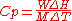where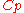= Specific heat at constant pressure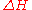= Enthalpy of solution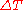= Change in temperature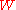= mass of solute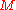= molecular mass of solute

## Differential scanning calorimeter

In a differential scanning calorimeter (DSC), heat flow into a sample—usually contained in a small aluminium
Aluminium
Aluminium or aluminum is a silvery white member of the boron group of chemical elements. It has the symbol Al, and its atomic number is 13. It is not soluble in water under normal circumstances....

capsule or 'pan'—is measured differentially, i.e., by comparing it to the flow into an empty reference pan.

In a heat flux
Heat flux
Heat flux or thermal flux is the rate of heat energy transfer through a given surface. The SI derived unit of heat rate is joule per second, or watt. Heat flux is the heat rate per unit area. In SI units, heat flux is measured in W/m2]. Heat rate is a scalar quantity, while heat flux is a vectorial...

DSC
, both pans sit on a small slab of material with a known (calibrated) heat resistance K. The temperature of the calorimeter is raised linearly with time (scanned), i.e., the heating rate
dT/dt = β
is kept constant. This time linearity requires good design and good (computerized) temperature control. Of course, controlled cooling and isothermal experiments are also possible.

Heat flows into the two pans by conduction. The flow of heat into the sample is larger because of its heat capacity
Heat capacity
Heat capacity , or thermal capacity, is the measurable physical quantity that characterizes the amount of heat required to change a substance's temperature by a given amount...

Cp. The difference in flow dq/dt induces a small temperature difference ΔT across the slab. This temperature difference is measured using a thermocouple
Thermocouple
A thermocouple is a device consisting of two different conductors that produce a voltage proportional to a temperature difference between either end of the pair of conductors. Thermocouples are a widely used type of temperature sensor for measurement and control and can also be used to convert a...

. The heat capacity can in principle be determined from this signal: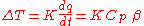Note that this formula (equivalent to Newton's law of heat flow) is analogous to, and much older than, Ohm's law
Ohm's law
Ohm's law states that the current through a conductor between two points is directly proportional to the potential difference across the two points...

of electric flow:
ΔV = R dQ/dt = R I.

When suddenly heat is absorbed by the sample (e.g., when the sample melts), the signal will respond and exhibit a peak.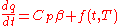From the integral
Integral
Integration is an important concept in mathematics and, together with its inverse, differentiation, is one of the two main operations in calculus...

of this peak the enthalpy of melting can be determined, and from its onset the melting temperature.

Differential scanning calorimetry is a workhorse technique in many fields, particularly in polymer
Polymer
A polymer is a large molecule composed of repeating structural units. These subunits are typically connected by covalent chemical bonds...

characterization.

A modulated temperature differential scanning calorimeter (MTDSC) is a type of DSC in which a small oscillation is imposed upon the otherwise linear heating rate.

This has a number of advantages. It facilitates the direct measurement of the heat capacity in one measurement, even in (quasi-)isothermal conditions. It permits the simultaneous measurement of heat effects that are reversible and not reversible at the timescale of the oscillation (reversing and non-reversing heat flow, respectively). It increases the sensitivity of the heat capacity measurement, allowing for scans at a slow underlying heating rate.

Safety Screening:- DSC may also be used as an initial safety screening tool. In this mode the sample will be housed in a non-reactive crucible (often Gold
Gold
Gold is a chemical element with the symbol Au and an atomic number of 79. Gold is a dense, soft, shiny, malleable and ductile metal. Pure gold has a bright yellow color and luster traditionally considered attractive, which it maintains without oxidizing in air or water. Chemically, gold is a...

, or Gold plated steel), and which will be able to withstand pressure
Pressure
Pressure is the force per unit area applied in a direction perpendicular to the surface of an object. Gauge pressure is the pressure relative to the local atmospheric or ambient pressure.- Definition :...

(typically up to 100 bar
Bar (unit)
The bar is a unit of pressure equal to 100 kilopascals, and roughly equal to the atmospheric pressure on Earth at sea level. Other units derived from the bar are the megabar , kilobar , decibar , centibar , and millibar...

). The presence of an exothermic
Exothermic
In thermodynamics, the term exothermic describes a process or reaction that releases energy from the system, usually in the form of heat, but also in the form of light , electricity , or sound...

event can then be used to assess the stability
Chemical stability
Chemical stability when used in the technical sense in chemistry, means thermodynamic stability of a chemical system.Thermodynamic stability occurs when a system is in its lowest energy state, or chemical equilibrium with its environment. This may be a dynamic equilibrium, where individual atoms...

of a substance to heat. However, due to a combination of relatively poor sensitivity, slower than normal scan rates (typically 2-3°/min - due to much heavier crucible) and unknonwn activation energy
Activation energy
In chemistry, activation energy is a term introduced in 1889 by the Swedish scientist Svante Arrhenius that is defined as the energy that must be overcome in order for a chemical reaction to occur. Activation energy may also be defined as the minimum energy required to start a chemical reaction...

, it is necessary to deduct about 75-100°C from the initial start of the observed exotherm to suggest a maximum temperature for the material. A much more accurate data set can be obtained from an adiabatic calorimeter, but such a test may take 2–3 days from ambient at a rate of 3°C increment per half hour.

## Isothermal titration calorimeter

In an isothermal titration
Titration
Titration, also known as titrimetry, is a common laboratory method of quantitative chemical analysis that is used to determine the unknown concentration of an identified analyte. Because volume measurements play a key role in titration, it is also known as volumetric analysis. A reagent, called the...

calorimeter
, the heat of reaction is used to follow a titration experiment. This permits determination of the mid point (stoichiometry
Stoichiometry
Stoichiometry is a branch of chemistry that deals with the relative quantities of reactants and products in chemical reactions. In a balanced chemical reaction, the relations among quantities of reactants and products typically form a ratio of whole numbers...

) (N) of a reaction as well as its enthalpy (delta H), entropy (delta S) and of primary concern the binding affinity (Ka)

The technique is gaining in importance particularly in the field of biochemistry
Biochemistry
Biochemistry, sometimes called biological chemistry, is the study of chemical processes in living organisms, including, but not limited to, living matter. Biochemistry governs all living organisms and living processes...

, because it facilitates determination of substrate binding to enzyme
Enzyme
Enzymes are proteins that catalyze chemical reactions. In enzymatic reactions, the molecules at the beginning of the process, called substrates, are converted into different molecules, called products. Almost all chemical reactions in a biological cell need enzymes in order to occur at rates...

s. The technique is commonly used in the pharmaceutical industry to characterize potential drug candidates.

## See also

• Enthalpy
Enthalpy
Enthalpy is a measure of the total energy of a thermodynamic system. It includes the internal energy, which is the energy required to create a system, and the amount of energy required to make room for it by displacing its environment and establishing its volume and pressure.Enthalpy is a...

• Heat
Heat
In physics and thermodynamics, heat is energy transferred from one body, region, or thermodynamic system to another due to thermal contact or thermal radiation when the systems are at different temperatures. It is often described as one of the fundamental processes of energy transfer between...

• Calorie
Calorie
The calorie is a pre-SI metric unit of energy. It was first defined by Nicolas Clément in 1824 as a unit of heat, entering French and English dictionaries between 1841 and 1867. In most fields its use is archaic, having been replaced by the SI unit of energy, the joule...

• Heat of combustion
Heat of combustion
The heat of combustion is the energy released as heat when a compound undergoes complete combustion with oxygen under standard conditions. The chemical reaction is typically a hydrocarbon reacting with oxygen to form carbon dioxide, water and heat...

• Calorimeter constant
Calorimeter constant
A calorimeter constant is a constant that quantifies the heat capacity of a calorimeter. It may be calculated by applying a known amount of heat to the calorimeter and measuring the calorimeter's corresponding change in temperature...

The source of this article is wikipedia, the free encyclopedia.  The text of this article is licensed under the GFDL.